## Investigation of iterative lensing templates

### Summary

Signal and noise maps of the iterative lensing templates are inferred for use in BK-style reanalysis. However, there are some problems which it seems might be resolvable by changing the reconstruction algorithm.

In this April 10 2020 posting Julien Carron presented new technology iterative lensing reconstructions. I plotted the cross correlation of these versus the ideal in this June 14 2020 posting. Julien's templates are provided for the 95GHz lensed-LCDM+noise maps only. It is apparently not possible to run the algorithm with signal only input maps while holding all else constant.

For the BK-style reanalysis we would like to know the noise component of the reconstructions, and the fraction of signal power which they retain versus the input sky.

Let's suppose the true sky has modes $$t_{lm}$$. We can attempt to model the modes of the reconstructed sky $$r_{lm}$$ as \begin{equation} r_{lm} = g_l t_{lm} + n_{lm} \end{equation} where $$g_l$$ is a gain factor (expected to be $$<1$$ due to Wiener filtering in the reconstruction), and $$n_{lm}$$ are the noise modes. (The above model assumes the filter is spatially uniform which is actually not the case - see below.)

Under the above model the cross spectra of the reconstructed and true modes is suppressed by one power of $$g_l$$ with respect to the input lensing power spectrum \begin{equation} g_l = \frac{C_l^{rt}}{C_l^{tt}}. \end{equation}

The auto spectrum of the reconstructed map is equal to \begin{equation} C_l^{rr} = g_l^2 C_l^{tt} + C_l^{nn}. \end{equation} Rearranging and substituting in for $$g_l$$ the noise bias is \begin{equation} C_l^{nn} = C_l^{rr} - \frac{(C_l^{rt})^2}{C_l^{tt}}. \end{equation}

Calculating $$C_l^{rr}$$, $$C_l^{tt}$$ and $$C_l^{rt}$$ for each of the currently available 100 realizations and taking the averages results in the plot below. Calculating the noise bias as above per realization and taking the mean is shown as the magenta line. The plot is shown twice with different x-axis ranges.

Figure 1 a and b: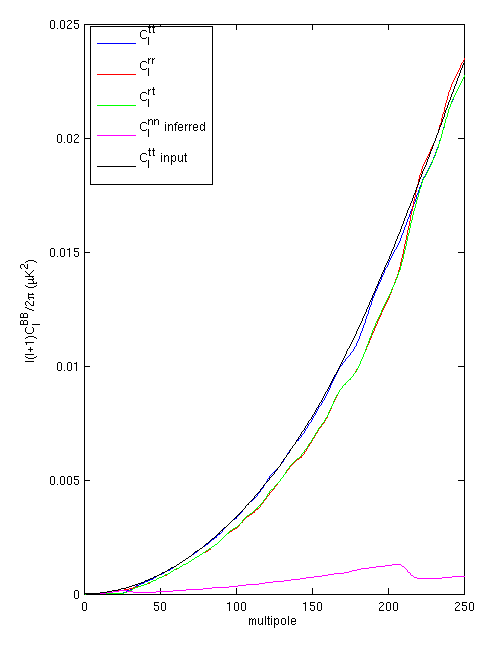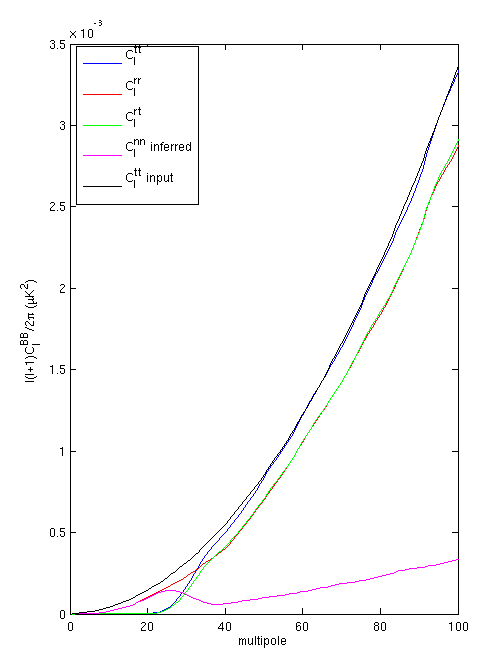We can also plot $$g_l$$ as below - again plot shown twice with different x-axis ranges.

Figure 2 a and b: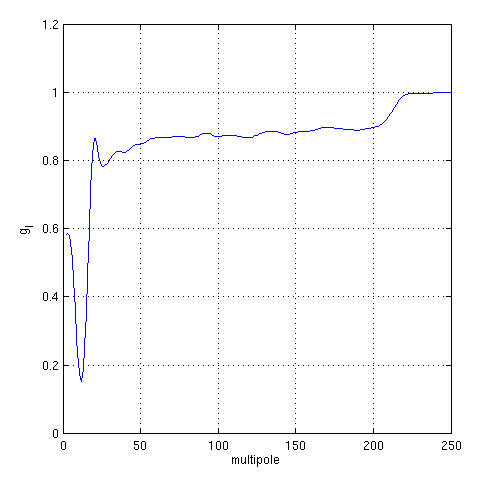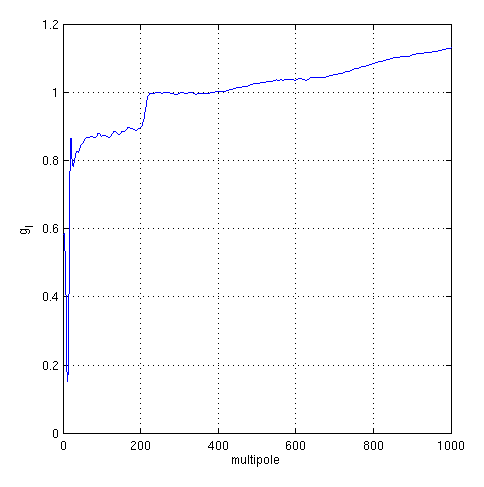[Details: 1) to go from maps to spectra above requires an apodization mask and I use the one selected for the 06 sim set in this post. 2) The $$t_{lm}$$ in the above are folded with a beam function (155GHz) which I divide out of the resulting spectra before using them.]

### Construction of noise and signal sim maps for BK style reanalysis

The above is a numerical investigation of the iterative lensing templates which Julien Carron has provided at the power spectrum level. The BK style reanalysis normally takes as input in each observing band sets of simulated maps of noise, signal and signal+noise, and takes power spectra of each of these. The mean of the noise spectra is subtracted from each of the signal+noise spectra. The ratio of the mean of the signal only spectra to the expectation values under the input sky model is taken as the "filter/beam supression factor". This is then divided out of each of the debiased signal+noise simulations to correct back to full power. The fluctuation of the fully corrected signal+noise spectra is then used to evaluate the constraining power of the data.

When adding the lensing template one may wish to remain as close to this traditional pattern as possible - and this what I am attempting to do in the following. I use the $$g_l$$ determined above plus equation 1 above to determine the noise $$a_{lm}$$'s. I take the B-mode $$t_{lm}$$ from the original lensed-LCDM input skies and the $$r_{lm}$$ which Julien provides to get the $$n_{lm}$$ on a per realization basis. We can also make maps of the $$g_l t_{lm}$$ as the signal simulations.

The plot below shows the $$Q$$ maps. The iterative reconstruction is at upper left, together with the inferred signal and noise components as described above at upper right and lower left. We see a problem immediately - clearly the iterative reconstruction includes a Wiener filter which is spatially varying. Therefore instead of increasing fluctuation towards the edge of the observed region it tends to zero. In all of the above I have assumed a single $$g_l$$ factor so my $$g_l t_{lm}$$ pattern remains constant amplitude to the edge. This means the inferred noise blows up around the edge. This happens in a normal experimental map but here it is happening for a different reason - because the model of equation 1 above is not a good one.

Figure 3: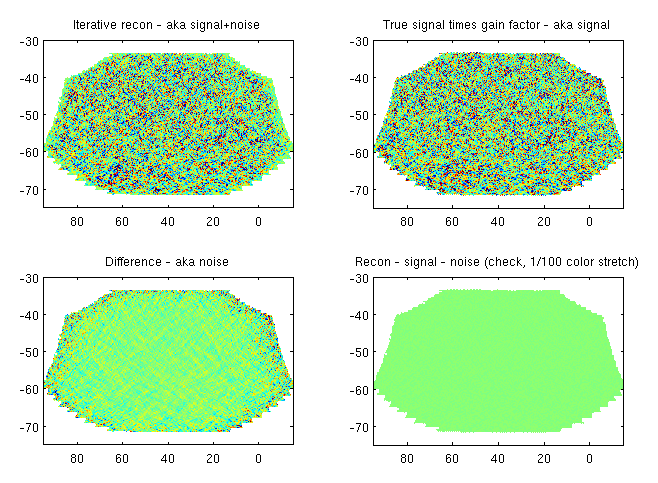I *think* using the above maps in the standard BK-style reanalysis pipeline will actually be OK so long as the apodization mask which is applied is the same one as I have used when measusing the $$g_l$$ factor. So long as the spectra we get back post apodization obey the usual rule mean of signal plus mean of noise equals mean of signal+noise, and the effective noise is correct, then I think it's all OK. We can test for the former as below and it seems to work.

Figure 4: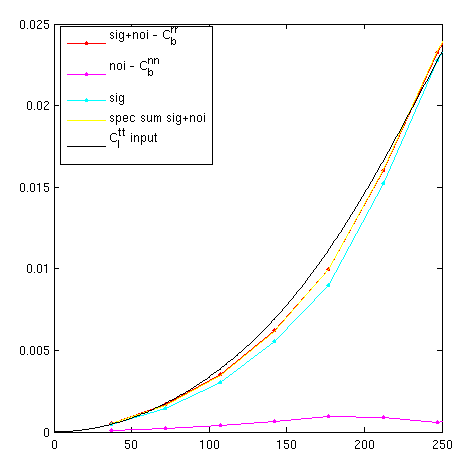However, the above begs the question as to whether the iterative reconstruction algorithm could be modified to deliver an output map where the signal component is not filtered - or has a constant degree of filtering over the full patch area. The noise would blow up around the edge but would get apodized down prior to taking the spectrum. Such maps would be more similar to the normal per band maps and - for the BK-style reanalysis at least would - would be better.